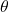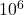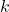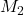# Method of moments

The method of moments is a simple idea for estimating the parameters of a model. Suppose the observed data are sampled iid from a distribution, our goal is to estimate the parameter. If we have enough data, we can get very close to the true mean of the distribution,, which is a  function of:. We know the form ofsince we know the form of.
For simple distributions, knowing just the mean is enough to invertto obtain. In general, we need to calculate the higher moments, which are also known functions of:. We then try to invert the systems of equationsto obtain. In practice, we typically only have enough data to accurately estimate the low order () moments.
A particularly cool application is that we can learn all the topics of a Latent Dirichlet Allocation (LDA) model using only the first three moments.  This sounds too good to be true, but a back of the envelope calculation shows that it’s actually reasonable. In LDA, each topic is a multinomial distribution over the vocabulary. So say the vocabulary is ~10,000 words and we want to estimate 100 topics, then we have ~ 1 million parameters. The third moments contains all probabilities of the form p(word1, word2, word3), where word1,2,3 can be any words in the vocabulary. Assuming we can estimate the third moments, then we havedata points to estimateparameters. Certainly plausible!
The method is quite intuitive. Suppose for simplicity we have an LDA model where each document has exactly one topic. Letdenote the (true) distribution for topic. The second momentis just thematrix (N=vocab size), where theentry is p(word_i, word_j). This can be written as a sum of outer products,. Similarly the third moment is a third order tensor, which generalizes the outer product,. The key point to notice is thatis a rank K tensor, whose eigenvectors are simply! Once we observe this, the rest of the algorithm comes down to using power iteration methods to recover the tensor eigenvectors, which give us the topic distributions. Very cool.